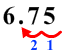# Multiplying Money

Rate Lesson 0 stars
Common Core
• Lesson size:
Message preview:
Someone you know has shared lesson with you:

To play this lesson, click on the link below:

https://www.turtlediary.com/lesson/multiplying-money.html

Hope you have a good experience with this site and recommend to your friends too.

Login to rate activities and track progress.
Login to rate activities and track progress.
CCSS.Math.Content.4.MD.A.2

Multiplying money is same as multiplying whole numbers.

Follow these steps to multiply money.

• When multiplying money, always put the larger number (the number with more digits) on top, and the smaller number (the number with less digits) on the bottom.
• Ignore the decimal point and multiply the numbers as we do with the whole numbers.
• Count how many total digits are on the right side of the decimal points in the numbers we are multiplying.
• Place the decimal point in the answer by starting at the right and moving a number of places equal to the sum of the decimal places in both numbers multiplied.

Let's go through few examples to understand better.

## Example 1

\$38.52 x 8

Ignore the decimal point and start by multiplying 3852 and 8.Now, count the number of digits to the right of the decimal point of both the numbers.

\$38.52 has two decimal places, while 8 has none. So, we have 2 decimal places in all .

Place the decimal point in the answer by starting at the right and moving 2 places to the right.\$38.52 x 8 = \$308.16

## Example 2

It costs \$1.35 for a slice of pizza, and Brandon wants to buy 5 slices for himself and his friend. How much will this cost?

Ignore the decimal point and start by multiplying 135 and 5.Now, count the number of digits to the right of the decimal point of both the numbers.

\$1.35 has two decimal places, while 5 has none. So, we have 2 decimal places in all .

Place the decimal point in the answer by starting at the right and moving 2 places to the right.So, the total cost of 5 slices of pizza is \$6.75

## Example 3

Veronica makes \$9.85 an hour at her new job. If she works 12 hours this week, how much money will she earn?

Ignore the decimal point and start by multiplying 985 and 12.Now, count the number of digits to the right of the decimal point of both the numbers.

\$9.85 has two decimal places, while 12 has none. So, we have 2 decimal places in all .

Place the decimal point in the answer by starting at the right and moving 2 places to the right.So, Veronica earns \$118.20 this week.

Summary
• Multiplying money is same as the multiplying whole numbers.
• Follow these steps to multiply money:
• When multiplying money, always put the larger number (the number with more digits) on top, and the smaller number (the number with less digits) on the bottom.
• Ignore the decimal point and multiply the numbers as we do with the whole numbers.
• Count how many total digits are on the right side of the decimal points in the numbers we are multiplying.
• Place the decimal point in the answer by starting at the right and moving a number of places equal to the sum of the decimal places in both numbers multiplied.

## More Money Lessons

Become premium member to get unlimited access.